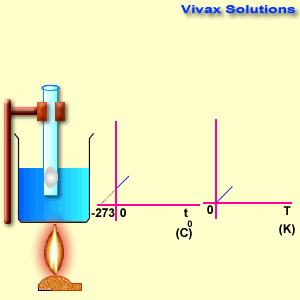# Charles Law

The volume of a fixed mass of gas is inversely proportional to its pressure at constant temperature.

The volume of a fixed mass of gas is directly proportional to its temperature at constant pressure.v ∝ t
v = k t
v = k t

As you can see, the line, when extended backwards crosses the x-axis at -273 C0. There is a special temperature when all gases have zero volume, called Absolute Temperature.
So, 0 T = -273 C
+ 273 => 273 T = 0C
+ t => (273 + t) T = t C
T = 273 + t, where t is the temperature in Celsius.

If the temperature and volume of a fixed mass of gas take the values of v1 T1and V2 T2 respectively, where T is absolute temperature,

V1 / T1 = V2 / T2

E.g.

The volume of a fixed mass of air is volume is 8 cm3 at 27 C0. Its temperature is raised to 127 C0, while keeping the pressure constant. Find the volume.
p1 = 20, v1 = 8, p2 = 40
According to Charles’s law,
V1 / T1 = V2 / T2
8 / (273 + 27) = v2 / (273 + 127)
v2 = 10.6cm3

http://www.vivaxsolutions.com/physics/alchrlslaw.aspx

This site uses Akismet to reduce spam. Learn how your comment data is processed.